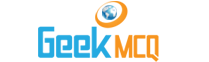Engineering :: Fluid Mechanics and Hydraulic Machines

1.  A perfect gas
 A. Has zero viscosity B. Has constant viscosity C. Satisfies the relation PV = mRT D. Is incompressible E. None of the above

2.  An ideal fluid is
 A. Similar to a perfect gas B. Frictionless and incompressible C. One which obeys Newton's law of viscosity D. One which satisfies continuity equation E. One which flows through pipes with least friction

3.  An ideal flow of any fluid must fulfil the following:
 A. Newton's law of viscosity B. Newton's law of motion C. Boundary layer theory D. Pascals law E. Continuity equation

4.  The velocity of a fluid particle at the centre of the pipe section is
 A. Maximum B. Minimum C. Average D. r.m.s. E. Logarithmic average.

5.  The shear stress velocity gradient relation of the Newtonian fluids is
 A. Linear B. Parabolic C. Hyperbolic D. Involutic E. There is no such relation

6.  The units of kinematic viscosity are
 A. kg/m2-sec B. kg sec/m2 C. m/kg sec D. m2/sec E. m kg/sec.

7.  The units of dynamic viscosity are
 A. Newton-sec/m2 B. Newton/m2-sec C. kg-sec/m D. kg m2/sec E. m2/sec

8.  The units of surface tension are
 A. Same as units of force B. Energy/unit area C. Force/unit area D. It is dimensionless E. None of the above

9.  Density in terms of viscosity is
 A. Kinematic viscosity/Dynamic viscosity B. Dynamic viscosity/Kinematic viscosity C. Kinematic viscosity x dynamic viscosity D. Any of the above E. None of the above

10.  Newton's law of viscosity relates
 A. Shear stress and rate of angular deformation in a fluid B. Yield shear stress, rate of angular deformation and viscosity C. Pressure, velocity and viscosity D. Shear stress, temperature, viscosity and velocity E. Pressure, vicosity and rate of angular deformation